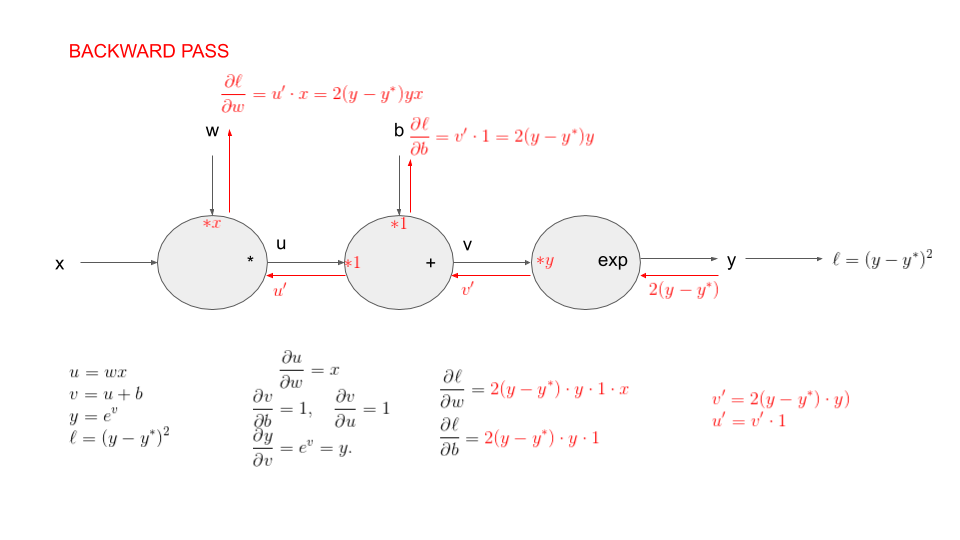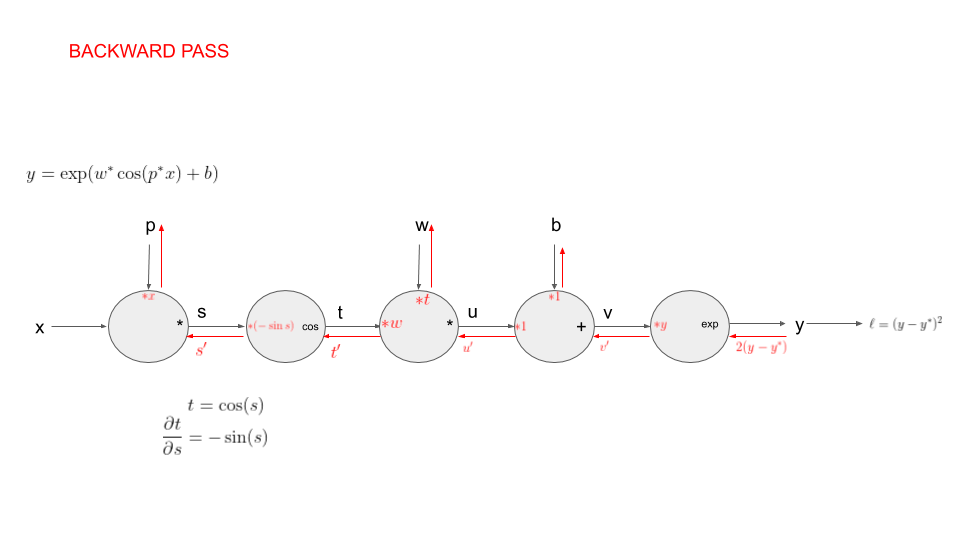# Module 2b - Automatic differentiation

## Automatic differentiation

0:00 Recap
0:40 A simple example (more in the practicals)
6:44 Pytorch backward function
9:05 The chain rule on our example
16:00 Linear regression
27:30 ... with pytorch tensors
34:35 Using a neural network (linear layer)
39:50 Using a pytorch optimizer
44:00 algorithm: how automatic differentiation works

## Quiz

To check your understanding of automatic differentiation, you can do the quizzes

## Practicals## Challenge• First modification: we now generate points $(x_t,y_t)$ where $y_t= \exp(w^*\cos(x_t)+b^*)$, i.e $y^*_t$ is obtained by applying a deterministic function to $x_t$ with parameters $w^*$ and $b^*$. Our goal is still to recover the parameters $w^*$ and $b^*$ from the observations $(x_t,y_t)$.
• Second modification: we now generate points $(x_t,y_t)$ where $y_t= \exp(w^*\cos(p^*x_t)+b^*)$, i.e $y^*_t$ is obtained by applying a deterministic function to $x_t$ with parameters $p^*$, $w^*$ and $b^*$. Our goal is still to recover the parameters from the observations $(x_t,y_t)$.